# numPy的一些知识点

Posted by kevin on February 13, 2020

## preface

numpy 早就用过了，但是长时间不用的话对其中的一些知识点又会忘记，又要去网上翻看各种博客，干脆自己把常用的一些东西记下来好了，以后忘了的话直接看自己写的笔记就行了

## numpy 基础

numpy 是 python 的矩阵运算库，底层由 C++ 编写，因此速度相比 python 自身快得多，经常用于数据科学领域中，语法和 Matlab 有些相似。

numpy（下面简称 np）的基本类型是 ndarray（n dimensions array），又用 np.array 称呼它，它有很多属性：np.ndim 表示数组的维度，np.size 表示数组中元素的个数，np.shape 表示数组各个维度的大小，例如一个三行四列矩阵的 shape 就是（3, 4），np.dtype 表示数组的数据类型，np 里面有很多的数据类型，如 np.int32，np.int16，np.float64，np.complex 等等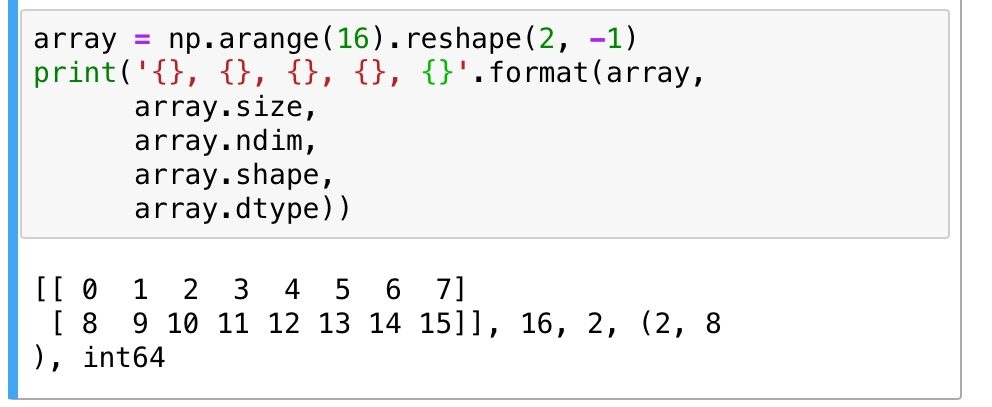### 创建 array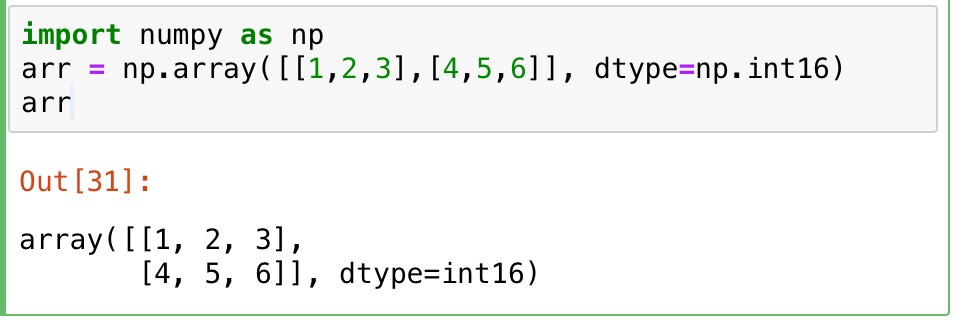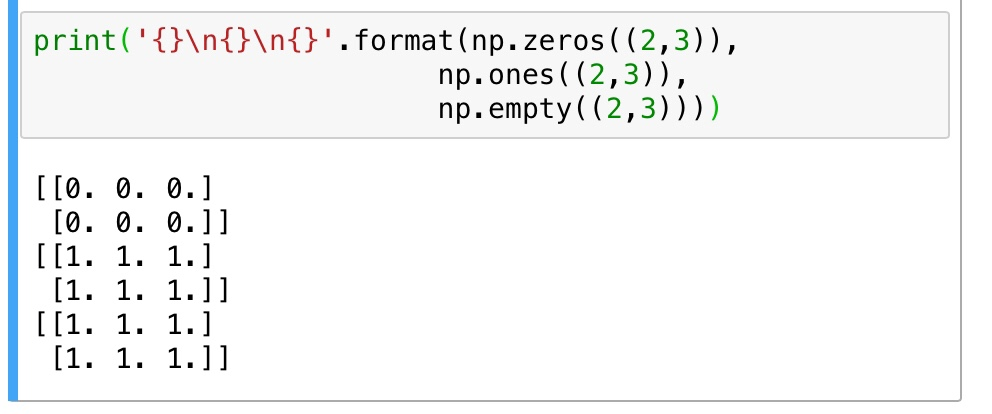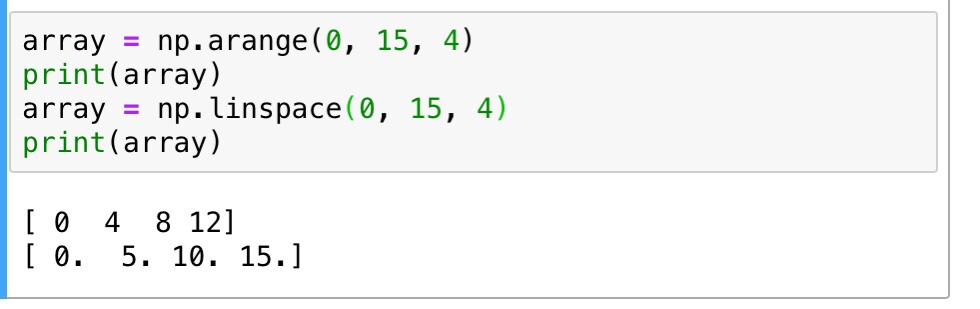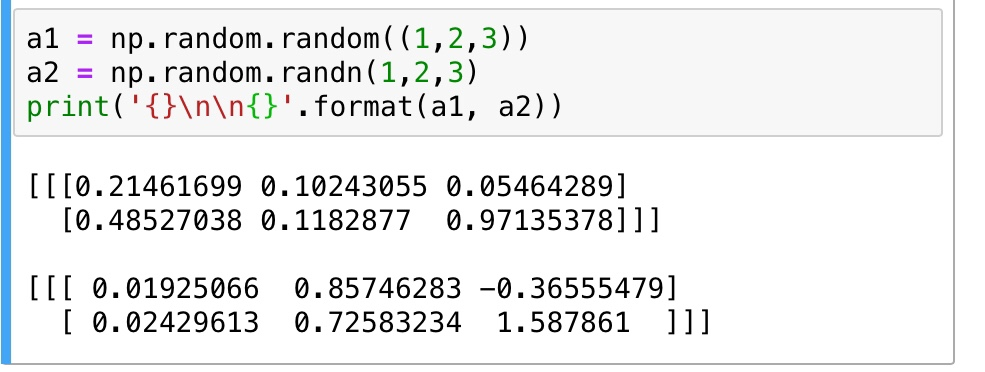### 基本运算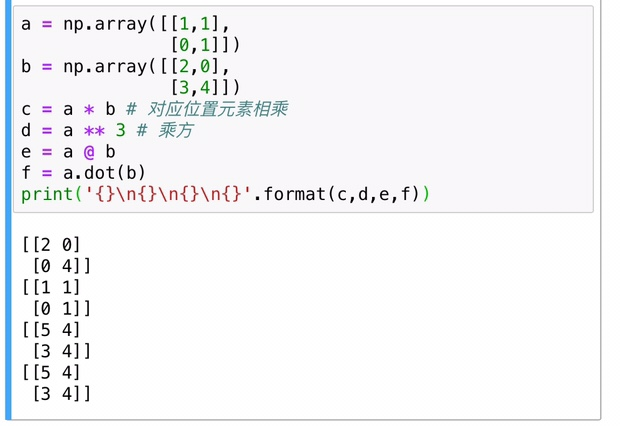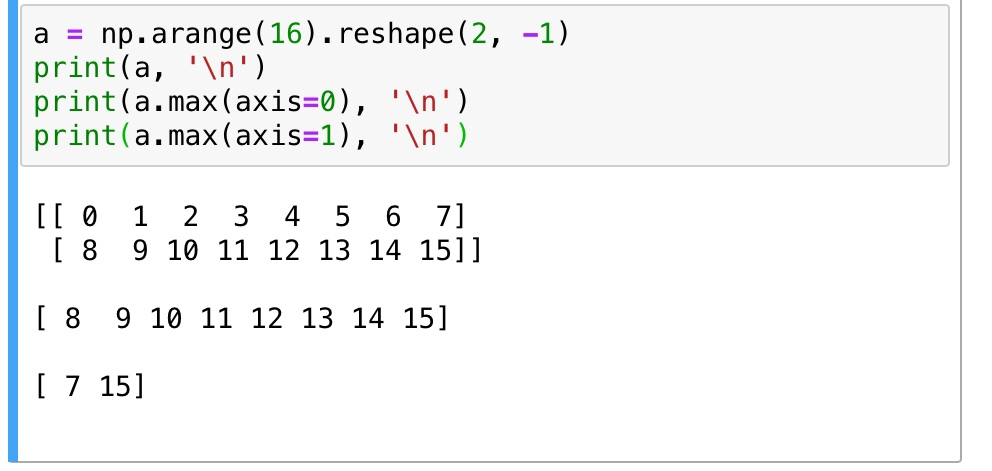## slice & index & iteration

np 的切片和索引和 python 是差不多的，甚至有些地方比 python 的还要更加高级一些，np 的多维矩阵的每个维度都可以运用切片，不同维度之间用逗号隔开，... 代表其他维度都按默认全部切片

x[1,2,…] is equivalent to x[1,2,:,:,:], x[…,3] to x[:,:,:,:,3] and x[4,…,5,:] to x[4,:,:,5,:]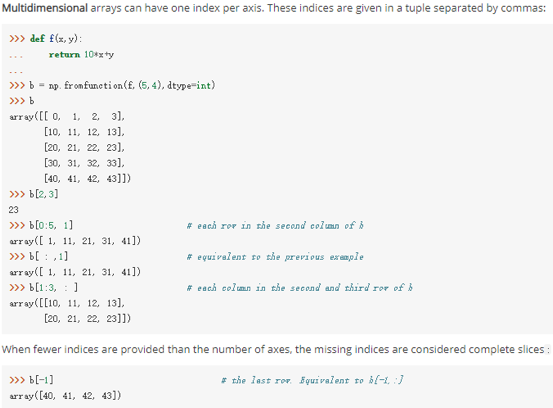np 的高级索引，不像 python 只能用字符和数字做索引，np 还可以用整形矩阵和布尔型矩阵来进行索引，虽然平时可能用的并不多。还可以用多维矩阵做索引，有点类似函数的感觉，看个例子就知道了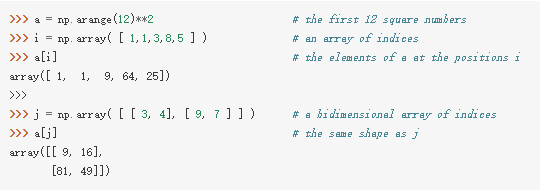## 改变 shape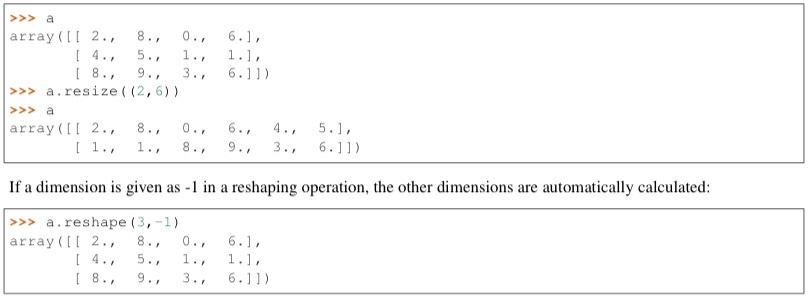### 堆叠和拆分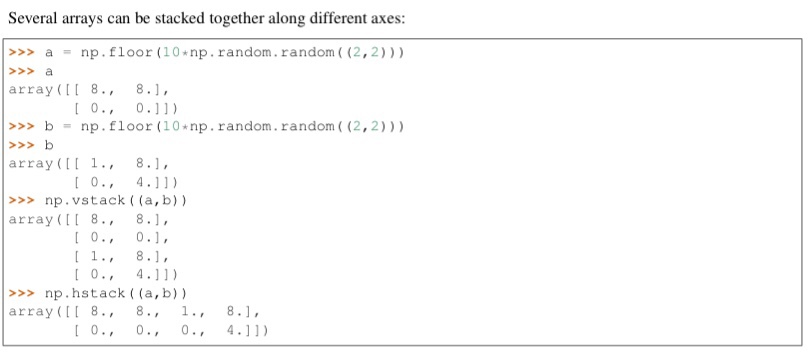## 深浅拷贝

np 中有点不同，如果直接将矩阵赋值给另一个矩阵，相当于没有拷贝，只是给矩阵换了个名字而已，因此如果有 a = b，b 改变的同时 a 也会改变。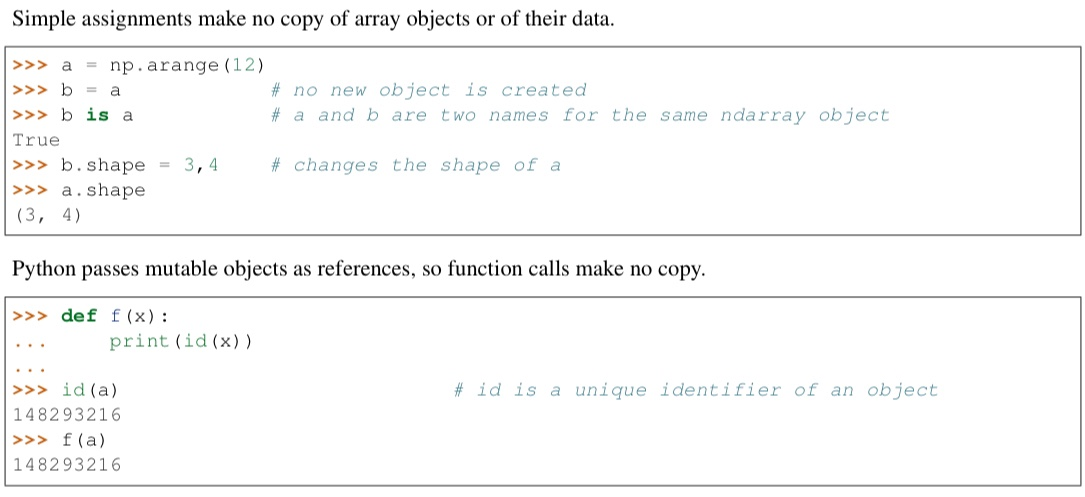np 的浅拷贝用 np.view() 来实现，view 只分享数据给另一个矩阵，b = a.view()，此时 b 就是 a 浅拷贝后的矩阵，a 改变 shape 后 b 的 shape 不改变，但是 a 改变数据的值后 b 的值也会改变。(值得注意的是 np 的切片返回的是原矩阵的浅拷贝，见下图中的例子)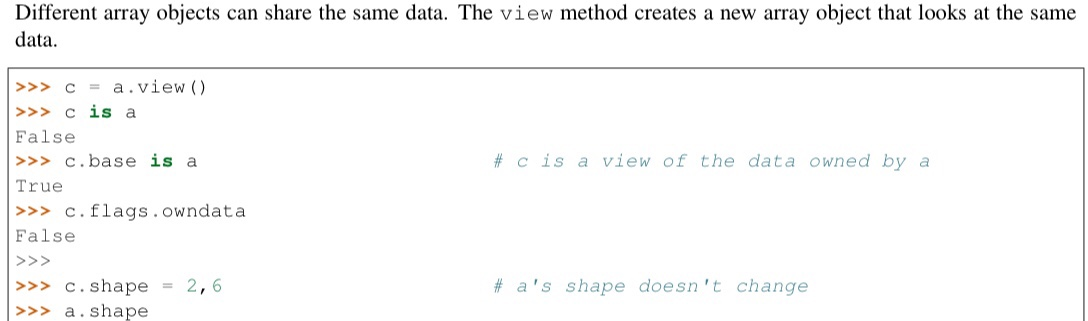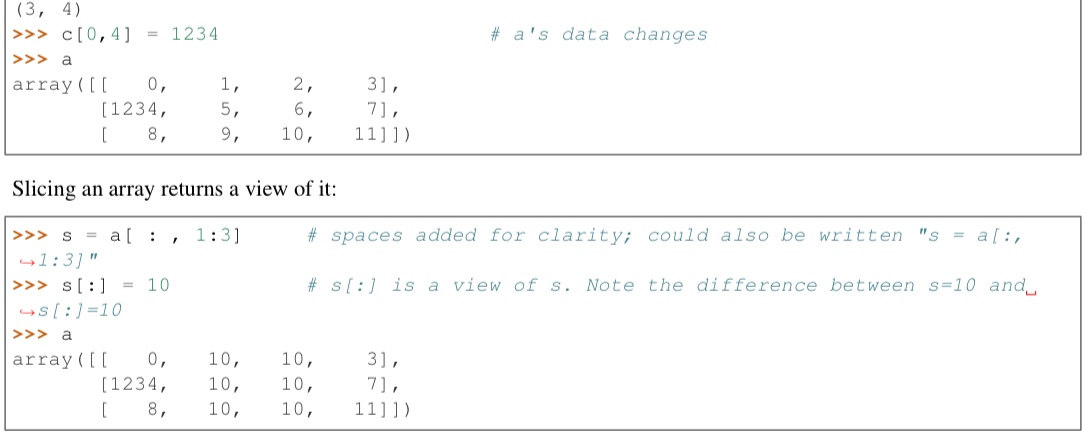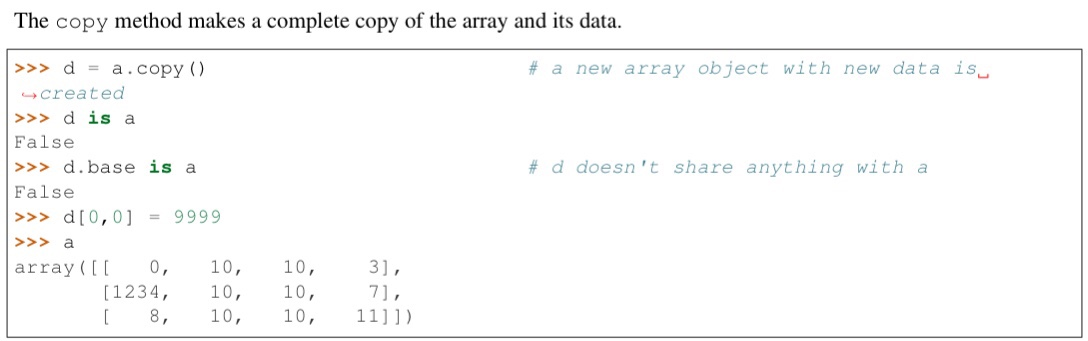## 线性代数

np 是为矩阵操作而设计的，所以里面也有很多的线性代数方面的接口，比如求逆 np.linalg.inv，求转置 np.transpose，创建单位阵 np.eye ，求矩阵的迹 np.trace，其实跟 matlab 里面的函数是差不多的，所以还是比较好掌握的，主要是理解线性代数中矩阵各种性质的原理。

## 广播

1. 两个数组各维度大小从后往前均一致（不够的维度就不用管）
2. 两个数组存在一些维度大小不相等时，有一个数组的该不相等维度大小为 1 （所以有些代码会用到很多增加一个维度的操作，就是为了能用广播）
A = np.zeros((2,5,3,4))
B = np.zeros((3,4))
print((A+B).shape)
print((A*B).shape)

A = np.zeros((4))
B = np.zeros((3,4))
print((A+B).shape)
print((A*B).shape)

A = np.zeros((2,5,3,4))
B = np.zeros((3,1))
print((A+B).shape)
print((A*B).shape)

A = np.zeros((2,5,3,4))
B = np.zeros((2,1,1,4))
print((A+B).shape)
print((A*B).shape)

A = np.zeros((1))
B = np.zeros((3,4))
print((A+B).shape)
print((A*B).shape)


(2, 5, 3, 4)
(2, 5, 3, 4)
(3, 4)
(3, 4)
(2, 5, 3, 4)
(2, 5, 3, 4)
(2, 5, 3, 4)
(2, 5, 3, 4)
(3, 4)
(3, 4)


## np.newaxis

# 一维情况
a = np.array([1,2,3,4,5])
a
array([1, 2, 3, 4, 5])
b = a[np.newaxis]
b
array([[1, 2, 3, 4, 5]])
# 多维情况
aa = np.array([[1,2,3,4],[4,5,6,7]])
aa
array([[1, 2, 3, 4],
[4, 5, 6, 7]])
bb = aa[np.newaxis]
bb
array([[[1, 2, 3, 4],
[4, 5, 6, 7]]])
aa.shape
(2, 4)
bb.shape
(1, 2, 4)
bb = aa[:,np.newaxis]
bb
array([[[1, 2, 3, 4]],
[[4, 5, 6, 7]]])
aa
array([[1, 2, 3, 4],
[4, 5, 6, 7]])
bb.shape
(2, 1, 4)
bb = aa[:,:,np.newaxis]
bb
array([[,
,
,
],
[,
,
,
]])


## np.meshgrid

simple-faster-rcnn 中有用到这个，记录一下。[X, Y] = meshgrid(x,y) 将向量 x 和 y 定义的区域转换成矩阵 X 和 Y , 其中矩阵 X 的行向量是向量 x 的简单复制，而矩阵 Y 的列向量是向量 y 的简单复制 (注：下面代码中 X 和 Y 均是数组，在文中统一称为矩阵了)。

import numpy as np

x = np.arange(0, 5)
print(x)
print('-------------')
y = np.arange(1, 3)
print(y)
print('-------------')
X, Y = np.meshgrid(x, y)
print(X)
print('-------------')
print(Y)
print('-------------')

[0 1 2 3 4]
-------------
[1 2]
-------------
[[0 1 2 3 4]
[0 1 2 3 4]]
-------------
[[1 1 1 1 1]
[2 2 2 2 2]]
-------------


reference: https://www.cnblogs.com/lemonbit/p/7593898.html

## np.argsort

argsort 函数返回的是数组值从小到大的索引值，也就是 index

>>> x = np.array([3, 1, 2])
>>> np.argsort(x)
array([1, 2, 0])
>>> x.argsort()
array([1, 2, 0])


>>> x = np.array([[0, 3], [2, 2]])
>>> np.argsort(x, axis=0) #按列排序
array([[0, 1],
[1, 0]])

>>> np.argsort(x, axis=1) #按行排序
array([[0, 1],
[0, 1]])


>>> x = np.array([3, 1, 2])
>>> np.argsort(-x)
array([0, 2, 1])


>>> x = np.array([3, 1, 2])
>>> np.argsort(x)[::-1]
array([0, 2, 1])


## np.append

annotations = np.zeros((0, 5))
annotation = np.zeros((1, 5))
annotation[0, :] = 1,2,3,4,5
annotations = np.append(annotations, annotation, axis=0)
annotations = np.append(annotations, annotation, axis=0)
annotations


array([[1., 2., 3., 4., 5.], [1., 2., 3., 4., 5.]])

## np.expand_dims

import numpy as np
a = np.array([1, 2, 3])
print(a.shape)
# a.shape(3,)

b = np.expand_dims(a, axis=0)
b = [[1, 2, 3]]
# b.shape = (1, 3)


## np.tile

numpy.tile(A, reps) 用来将 A 的元素重复 reps 次，类似与 numpy 的广播机制，不过文档强烈建议直接用广播机制不要用这个函数

>>> c = np.array([1,2,3,4])
>>> np.tile(c, 4)
array([1, 2, 3, 4, 1, 2, 3, 4, 1, 2, 3, 4, 1, 2, 3, 4])
>>> np.tile(c, (4,1))
array([[1, 2, 3, 4],
[1, 2, 3, 4],
[1, 2, 3, 4],
[1, 2, 3, 4]])


## np.dot 和 np.matmul

>>> import numpy as np
>>> a = np.array([[1,2,3],[4,5,6]])
>>> b = np.array([[1,2],[3,4],[5,6]])
>>> np.matmul(a,b)
array([[22, 28],
[49, 64]])
>>> np.dot(a,b)
array([[22, 28],
[49, 64]])


## np.linalg.norm

norm 表示范数，这个 api 用来求范数 np.linalg.norm(x, ord=None, axis=None, keepdims=False)。默认情况下求的是二范数，也就是对应位置元素相乘再相加再开方

axis：处理类型

axis=1表示按行向量处理，求多个行向量的范数

axis=0表示按列向量处理，求多个列向量的范数

axis=None表示矩阵范数

keepding：是否保持矩阵的二维特性

True表示保持矩阵的二维特性，False相反

import numpy as np
x = np.array([
[0, 3, 4],
[1, 6, 4]])
#默认参数ord=None，axis=None，keepdims=False
print("默认参数(矩阵整体元素平方和开根号，不保留矩阵二维特性)：",np.linalg.norm(x))
print("矩阵整体元素平方和开根号，保留矩阵二维特性：",np.linalg.norm(x,keepdims=True))

print("矩阵每个行向量求向量的2范数：",np.linalg.norm(x,axis=1,keepdims=True))
print("矩阵每个列向量求向量的2范数：",np.linalg.norm(x,axis=0,keepdims=True))

print("矩阵1范数：",np.linalg.norm(x,ord=1,keepdims=True))
print("矩阵2范数：",np.linalg.norm(x,ord=2,keepdims=True))
print("矩阵∞范数：",np.linalg.norm(x,ord=np.inf,keepdims=True))

print("矩阵每个行向量求向量的1范数：",np.linalg.norm(x,ord=1,axis=1,keepdims=True))


## np.transpose

vis_img = np.transpose(a, (1,2,0))